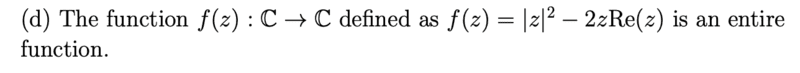# True or false? |z|^2 is an entire function

docnet
Homework Statement:
.
Relevant Equations:
.False

The absolute value function is is not analytic wherever its argument equals zero. ##f## is not analytic at ##z=0## so it is not entire.

Homework Helper
Gold Member
2022 Award
Homework Statement:: .
Relevant Equations:: .

View attachment 294651

False

The absolute value function is is not analytic wherever its argument equals zero. ##f## is not analytic at ##z=0## so it is not entire.
That's a fairly significant logical fallacy!

In general, if ##f(z) = f_1(z) + f_2(z)##, then even if ##f_1## and ##f_2## are not analytic, their sum may be. Intuitively, the non-analytic behaviour of ##f_1## and ##f_2## may cancel each other out in some way.

••docnet and S.G. Janssens
What can you say about the zeros of ##f## in the complex plane?

•docnet
docnet
That's a fairly significant logical fallacy!

In general, if ##f(z) = f_1(z) + f_2(z)##, then even if ##f_1## and ##f_2## are not analytic, their sum may be. Intuitively, the non-analytic behaviour of ##f_1## and ##f_2## may cancel each other out in some way.
really? that is surprising to me.

Homework Helper
Gold Member
2022 Award
Take as an example: $$f(z) = z = Re(z) +iIm(z)$$ is the sum of two non-analytic functions!

•docnet
docnet
What can you say about the zeros of ##f## in the complex plane?
the zeroes of ##f## are given by $$|z|^2=2zRe(z)$$
the left size is real valued, so the right side has to be real valued. The equation ##x^2=2x^2## has no solutions other than ##x=0##, so the zero of ##f## is ##z=0##.

Gold Member
You have one thing a bit backwards: ##z=0## is actually the only point where ##|z|^2## is complex differentiable. You can verify this from the Cauchy Riemann equations.

And anyway, a function ##f## being (not) differentiable at a point doesn't mean the same is true for ##f+g.##

For your function, you can also write it in real and imaginary parts and see whether the Cauchy-Riemann equations hold.

Edit: Apologies, while I was writing this, several posts appeared that i didn't notice!

•docnet
docnet
Take as an example: $$f(z) = z = Re(z) +iIm(z)$$ is the sum of two non-analytic functions!
okay.. that makes sense

docnet
You have one thing a bit backwards: ##z=0## is actually the only point where ##|z|^2## is complex differentiable. You can verify this from the Cauchy Riemann equations.

And anyway, a function ##f## being (not) differentiable at a point doesn't mean the same is true for ##f+g.##

For your function, you can also write it in real and imaginary parts and see whether the Cauchy-Riemann equations hold.
How do you tell the real and imaginary parts of ##z## in the absolute value function? this is what I wanted to do, compute the Cauchy Riemann equations, but the absolute value function confused me.

Homework Helper
Gold Member
2022 Award
okay.. that makes sense
You know what I'm going to say ... you did the same thing again! You jumped at an easy, one-line proof, that you didn't seriously question. You must be able to fully justify each step you take.

Developing your maths skills means developing the ability to find the example I gave in post #5.

•docnet
docnet
How do you tell the real and imaginary parts of ##z## in the absolute value function? this is what I wanted to do, compute the Cauchy Riemann equations, but the absolute value function confused me.
Oh gosh.. I forgot that the absolute value function has a formal definition ##|z|=\sqrt{z\bar{z}}##. I'm so sorry @PeroK

Homework Helper
Gold Member
2022 Award
How do you tell the real and imaginary parts of ##z## in the absolute value function? this is what I wanted to do, compute the Cauchy Riemann equations, but the absolute value function confused me.
The absolute value is real, it has no imaginary part.

•docnet
Gold Member
How do you tell the real and imaginary parts of in the value funciton? this is what I wanted to do, compute the Cauchy Riemann equations, but the absolute value funciton confused me.
You would write ##z=x+iy## (so ##\text{Re}(z)=x## and ##|z|^2=x^2+y^2##).

••docnet and PeroK
docnet
$$f=|z|^2-2zRe(z)=x^2+y^2-2(x+iy)x$$
$$=(y^2-x^2)-i(2xy)$$
$$v_x=-2x=u_y$$
$$-v_y=-2y=u_x$$
so ##f=|z|^2-2zRe(z)## satisfies the Cauchy Riemann equations and so it's entire.

•PeroK
Homework Helper
Gold Member
2022 Award
so ##f=|z|^2-2zRe(z)## satisfies the Cauchy Riemann equations and so it's entire.
Would you believe it!

•docnet
docnet
There goes my dreams for a Ph.D in maths, smashed to granules.

You'd certainly bash out a PhD thesis quickly enough. Whether it would be defensible is another matter!•